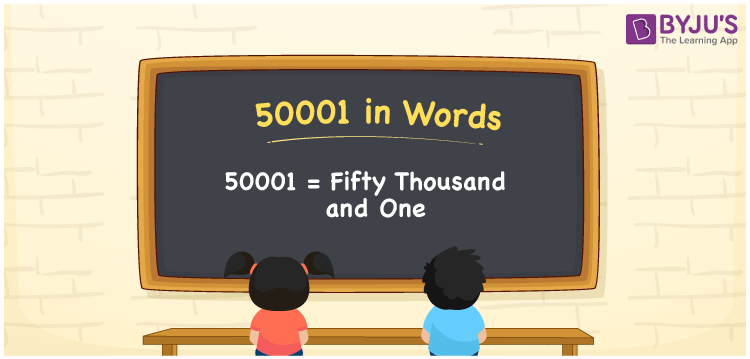# 50001 in Words

50001 in words can be written as Fifty Thousand and One. The fundamental concepts in Mathematics like counting or count can be learnt efficiently here. If you buy a bike for Rs. 50001, then you can say that “I bought a bike for Fifty Thousand and One Rupees”. To write numbers in words, the English alphabet is used. The numbers in words concept is explained here in a simple way to improve the conceptual knowledge of students. The 50001 can be read as “Fifty Thousand and One” in English.

 50001 in words Fifty Thousand and One Fifty Thousand and One in Numbers 50001

## 50001 in English Words## How to Write 50001 in Words?

Students can learn about the expanded form and the place value chart of 50001. Five digits are present in the number 50001. With the help of the place value chart given below, students will be able to understand the concepts with ease.

 Ten Thousands Thousands Hundreds Tens Ones 5 0 0 0 1

50001 can be written in expanded form as:

5 x Ten Thousand + 0 x Thousand + 0 × Hundred + 0 × Ten + 1 × One

= 5 x 10000 + 0 x 1000 + 0 × 100 + 0 × 10 + 1 × 1

= 50000 + 1

= 50001

= Fifty Thousand and One

Hence, 50001 in words is written as Fifty Thousand and One.

50001 is a natural number that precedes 50002 and succeeds 50000.

50001 in words – Fifty Thousand and One

Is 50001 an odd number? – Yes

Is 50001 an even number? – No

Is 50001 a perfect square number? – No

Is 50001 a perfect cube number? – No

Is 50001 a prime number? – No

Is 50001 a composite number? – Yes

## Frequently Asked Questions on 50001 in Words

Q1

### How to write 50001 in words?

50001 can be written in words as “Fifty Thousand and One”.
Q2

### How to write Fifty Thousand and One in numbers?

Fifty Thousand and One in numbers can be written as 50001.
Q3

### Is 50001 an odd or even number?

50001 is an odd number as it is not completely divisible by 2.